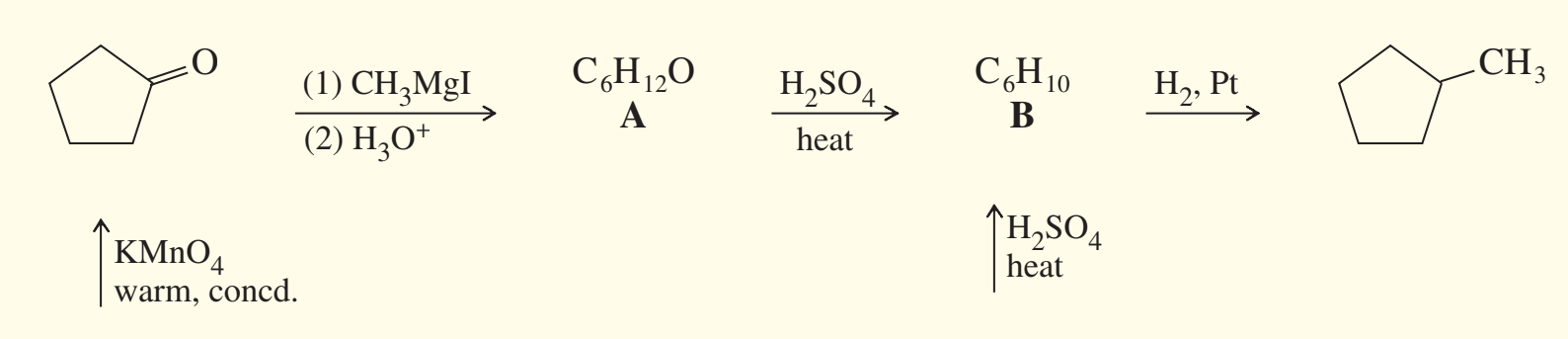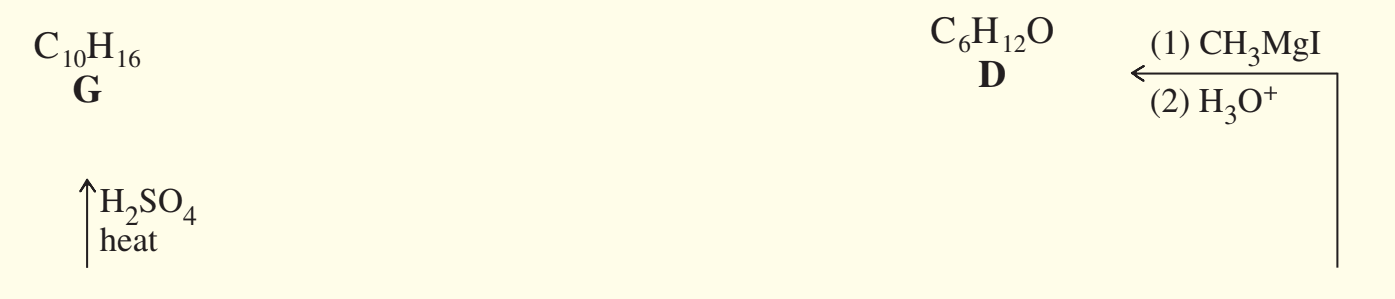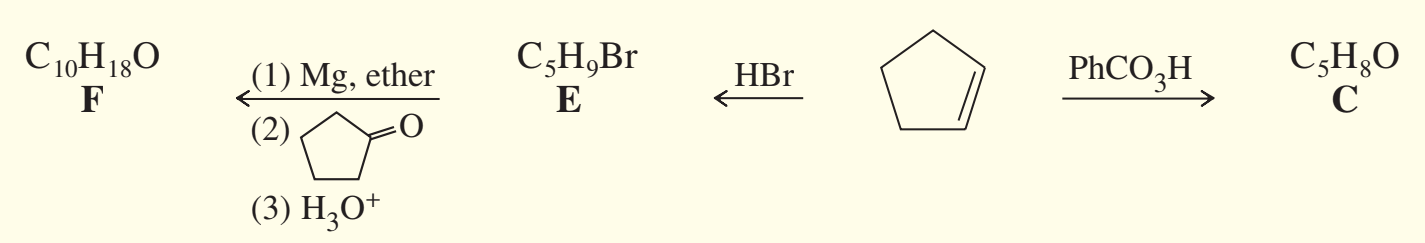×
Get Full Access to Organic Chemistry - 8 Edition - Chapter 10 - Problem 49sp
Get Full Access to Organic Chemistry - 8 Edition - Chapter 10 - Problem 49sp

×

# Determine the structures of compounds A through G,ISBN: 9780321768414 33

## Solution for problem 49SP Chapter 10

Organic Chemistry | 8th Edition

• Textbook Solutions
• 2901 Step-by-step solutions solved by professors and subject experts
• Get 24/7 help from StudySoup virtual teaching assistantsOrganic Chemistry | 8th Edition

4 5 1 279 Reviews
31
1
Problem 49SP

Determine the structures of compounds A through G, including stereochemistry where appropriate.Step-by-Step Solution:

Solution 49SP

Step 1Step 2 of 2

## Discover and learn what students are asking

Calculus: Early Transcendental Functions : Graphs and Models
?In Exercises 5-14, sketch the graph of the equation by point plotting. $$y=4-x^{2}$$

Chemistry: The Central Science : Molecular Geometry and Bonding Theories
?Give approximate values for the indicated bond angles in the following molecules:

Statistics: Informed Decisions Using Data : Data Collection
?In Problems 1–5, provide a definition using your own words. 3. Sample

Statistics: Informed Decisions Using Data : Data Collection
?List and describe the three major types of observational studies.

Statistics: Informed Decisions Using Data : Estimating a Population Standard Deviation
?True or False: The chi-square distribution is symmetric.

Unlock Textbook Solution